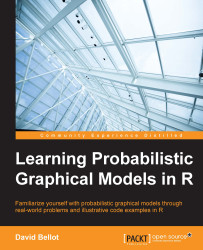•#### Learning Probabilistic Graphical Models in R#### Overview of this book

Probabilistic graphical models (PGM, also known as graphical models) are a marriage between probability theory and graph theory. Generally, PGMs use a graph-based representation. Two branches of graphical representations of distributions are commonly used, namely Bayesian networks and Markov networks. R has many packages to implement graphical models. We’ll start by showing you how to transform a classical statistical model into a modern PGM and then look at how to do exact inference in graphical models. Proceeding, we’ll introduce you to many modern R packages that will help you to perform inference on the models. We will then run a Bayesian linear regression and you’ll see the advantage of going probabilistic when you want to do prediction. Next, you’ll master using R packages and implementing its techniques. Finally, you’ll be presented with machine learning applications that have a direct impact in many fields. Here, we’ll cover clustering and the discovery of hidden information in big data, as well as two important methods, PCA and ICA, to reduce the size of big problems.
Learning Probabilistic Graphical Models in RCreditswww.PacktPub.comPrefaceFree Chapter
Probabilistic ReasoningExact InferenceLearning ParametersBayesian Modeling – Basic ModelsApproximate InferenceBayesian Modeling – Linear ModelsProbabilistic Mixture ModelsAppendixIndex## The Gaussian mixture model

The Gaussian mixture model is the first example of a latent variable model. Latent variables are also called hidden variables and are variables that are present in the model but are never observed.

The notion of using unobserved variables can be surprising at first because we might wonder how to estimate the parameters of the distribution of such a variable. In fact, we might wonder what the real meaning of such a latent variable is.

For example, let's say we observe data represented by a group of random variables. This data tends to group into clusters, aggregating together depending on their underlying meaning. For example, we could observe physiological traits from animals and group those data points by species such as dogs, cats, cows, and so on. If we think in terms of generating models, then we can say that, by choosing a group such as for example pony, we will observe features that are specific to this group and not to another group such as cats. However,...